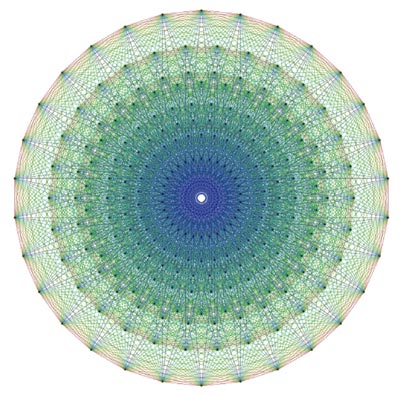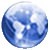﻿ E8 PhysicsVertex-edge graph of the E8 polytope  An Exceptionally Simple Theory of Everything Edited by Andy Ross Garrett Lisi, 39, completed his doctorate in theoretical physics in 1999 at the University of California, San Diego, but has no university affiliation and spends most of the year surfing in Hawaii. In winter, he heads to Nevada, where he snowboards.His proposal is remarkable because it does not require highly complex mathematics, it does not require more than one dimension of time and three of space, and it may even be testable, since it predicts a host of new particles.Lee Smolin at the Perimeter Institute for Theoretical Physics in Waterloo, Ontario, Canada, describes Lisi's work as "fabulous". "It is one of the most compelling unification models I've seen in many, many years," he says."Some incredibly beautiful stuff falls out of Lisi's theory," adds David Ritz Finkelstein at the Georgia Institute of Technology, Atlanta. "This must be more than coincidence and he really is touching on something profound."He has high hopes that his new theory could provide a radical new explanation for the Standard Model, which weaves together three of the four fundamental forces of nature: the electromagnetic force, the strong force, and the weak force. His model also takes account of gravity.Lisi's inspiration lies in the most elegant and intricate shape known to mathematics, called E8, only fully understood by mathematicians earlier this year. E8 is at the heart of many bits of physics. One interpretation of why we have such a quirky list of fundamental particles is because they all result from different facets of the symmetries of E8.Lisi realised that he could find a way to place the various elementary particles and forces on the 248 points of E8. What remained was 20 gaps which he filled with notional particles, for example those that some physicists predict to be associated with gravity.Physicists have long puzzled over why elementary particles appear to belong to families, but this arises naturally from the geometry of E8, he says. So far, all the interactions predicted by the complex geometrical relationships inside E8 match with observations in the real world. "How cool is that?" he says.The crucial test of Lisi's work will come only when he has made testable predictions. Lisi is now calculating the masses that the 20 new particles should have, in the hope that they may be spotted when the Large Hadron Collider starts up.  Is this the fabric of the universe? Edited by Andy Ross Mathematicians today unveiled the result of an arcane investigation of a group called E8. The combined assault on what is described as "one of the largest and most complicated structures in mathematics" required the effort of 18 mathematicians from America and Europe for an intensive four-year collaboration."The group of symmetries of this strange geometry called E8 is one of the most intriguing structures that Nature has left for the mathematician to play with," commented Professor Marcus du Sautoy of Oxford University. "Most of the time mathematical objects fit into nice patterns that we can order and classify. But this one just sits there like a huge Everest."For the feat, the team used a mix of theoretical mathematics and intricate computer programming to map E8, which is an example of a Lie group. Lie groups were invented by the 19th century Norwegian mathematician Sophus Lie to study symmetry. Underlying any symmetrical object is a Lie group."E8 was discovered over a century ago, in 1887, and until now, no one thought the structure could ever be understood," said Professor Jeffrey Adams, Project Leader, at the University of Maryland. "This groundbreaking achievement is significant both as an advance in basic knowledge, as well as a major advance in the use of large scale computing to solve complicated mathematical problems.""This is an exciting breakthrough," said Professor Peter Sarnak at Princeton University. "Understanding and classifying the representations of E8 and Lie groups has been critical to understanding phenomena in many different areas of mathematics and science including algebra, geometry, number theory, physics and chemistry."The goal was to describe all the possible representations of E8. These representations are extremely complicated, but mathematicians describe them in terms of basic building blocks. The new result is a complete list of these building blocks for the representations of E8, and a precise description of the relations between them, all encoded in a matrix, or grid, with 453,060 rows and columns. In all, there are 205,263,363,600 entries, each one a polynomial.The result of the E8 calculation, which contains all the information about E8 and its representations, is 60 gigabytes in size. The computation required sophisticated new mathematical techniques and computing power not available even a few years ago."This is an impressive achievement," said Hermann Nicolai, Director of the Albert Einstein Institute in Potsdam, Germany.  E8 Edited by Andy Ross In mathematics, E8 is the name given to a family of closely related structures. In particular, it is the name of some exceptional simple Lie algebras as well as that of the associated simple Lie groups. It is also the name given to the corresponding root system, root lattice, and various related groups. E8 was formulated between the years of 1888 and 1890 by Wilhelm Killing.The designation E8 comes from Wilhelm Killing and Élie Cartan's classification of the complex simple Lie algebras, which fall into four infinite families and five exceptional cases. The E8 algebra is the largest and most complicated of these exceptional cases.E8 has rank 8 (the maximum number of mutually commutative degrees of freedom) and dimension 248 (as a manifold). The vectors of the root system are in eight dimensions. The Weyl group of E8, which acts as a symmetry group of the maximal torus by means of the conjugation operation from the whole group, is of order 696,729,600.E8 is unique among simple Lie groups in that its non-trivial representation of smallest dimension is the adjoint representation (of dimension 248) acting on the Lie algebra E8 itself. The complex Lie group E8 of complex dimension 248 can be considered as a simple real Lie group of (real) dimension 496, which is simply connected, has maximal compact subgroup the compact form of E8, and has an outer automorphism group of order 2 generated by complex conjugation. As well as the complex Lie group of type E8, there are three real forms of the group, all of real dimension 248.The coefficients of the character formulas for infinite dimensional irreducible representations of E8 depend on some large square matrices consisting of polynomials. These matrices were computed after four years of collaboration by a group of 18 mathematicians and computer scientists, led by Jeffrey Adams, with much of the programming done by Fokko du Cloux. The result was announced in March 2007.The compact real form of E8 is the isometry group of a 128-dimensional Riemannian manifold known informally as the octo-octonionic projective plane because it can be built using an algebra that is the tensor product of the octonions with themselves.A root system of rank r is a particular finite configuration of vectors, called roots, which span an r-dimensional Euclidean space and satisfy certain geometrical properties. In particular, the root system must be invariant under reflection through the hyperplane perpendicular to any root.The E8 root system is a rank 8 root system containing 240 root vectors spanning R8. It is irreducible in the sense that it cannot be built from root systems of smaller rank. Each of the root vectors in E8 have equal length.The E8 Lie group has applications in string theory and supergravity. The group E8 x E8 (the Cartesian product of two copies of E8) serves as the gauge group of one of the two types of heterotic string and is one of two anomaly-free gauge groups that can be coupled to N = 1 supergravity in 10 dimensions. E8 is the U-duality group of supergravity on an eight-torus (in its split form).One way to incorporate the standard model of particle physics into heterotic string theory is the symmetry breaking of E8 to its maximal subalgebra SU(3) x E6. In 1982, Michael Freedman used the E8 lattice to construct an example of a topological 4-manifold, the E8 manifold, which has no smooth structure.  An Exceptionally Simple Theory of Everything This theory claims to unify all fields of the standard model with gravity using a 248-point lattice of E8 geometry. The title is a mathematical pun on E8's classification as both a simple group and an exceptional group. In its current form, the theory does not reproduce the particle content of the Standard Model (SM) and it is not a quantum theory. Lisi claims that it will be testable soon using the Large Hadron Collider which is slated to come on line in May 2008. However, his paper contains no calculations for particle masses, and it is not clear that such calculations could be done even in principle.Sabine Hossenfelder, of the Perimeter Institute for Theoretical Physics, said the model:— Does not naturally lead to a unification of the SM interactions with gravity (he has to choose the action by hand that contains both).— Does not allow us to understand quantum gravity (since nothing is said about quantization).— Does not explain the parameters in the SM (it does not yet have a mechanism for symmetry breaking).— Does not explain the cosmological constant or its value.— Does not explain the hierarchy problem.— Does not explain why we live in a spacetime with 4 dimensions.Overall she thinks the paper has potential. AR  This theory animated me greatly in 2008.